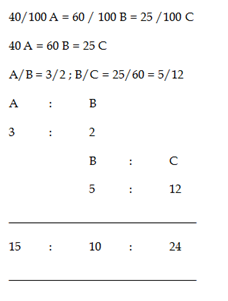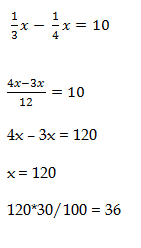# RRB ALP 2018 Practice Test Papers | Arithmetic Questions (Day-85)

Dear Aspirants, Here we have given the Important RRB ALP & Technicians Exam 2018 Practice Test Papers. Candidates those who are preparing for RRB ALP 2018 can practice these Arithmetic Questions to get more confidence to Crack RRB 2018 Examination.

[WpProQuiz 3212]

Click “Start Quiz” to attend these Questions and view Solutions

1) The difference between the discount of 40% and two successive discounts of 20 % is what percentage?

a) 6 %

b) 11 %

c) 3 1/3 %

d) 4 %

2) A and B can do a piece of work in 10 days. B and C can do that work in 15 days. C and A can do that in 12 days. How many days C needed to finish the work alone?

a) 20 days

b) 30 days

c) 40 days

d) 18 days

3) 40% of A = 60 % of B = 25 % of C. What is the ratio of A : B : C?

a) 24 : 25 : 15

b) 25 : 20 : 12

c) 15 : 10 : 24

d) 10 : 12 : 15

4) In an examination there are 100 boys and 200 girls, who are passed only 56% of boys and 72% of girls. What is the percentage of failed boys less than that of girls?

a) 17 ½

b) 19 ¾

c) 20 ½

d) 21 3/ 7

5) 5625 + 15129 = ?

a) 201

b) 245

c) 213

d) 198

6) Find the value of cosec 60°. cos30 °

a) 0

b) -1

c) 1

d) 2

7) A’s age is double the time that of B’s age and tripled the time of C’s age’. If Difference of A’s age and C’s age is 8. What is the age of B?

a) 15

b) 23

c) 14

d) 6

8) If a man is travelled 30 km in 5 kmph, he will be late by 30 mins. If he travelled the same distance in 6 kmph, how early from the fixed time will he reach?

a) 40 mins

b) 30 mins

c) 20 mins

d) 10 mins

9) 1/3rd of a number exceeds ¼th of same number by 10. Find the 30% of the number

a) 40

b) 42

c) 36

d) 48

10) 1/3rd of ¾ of a number is

a) ½ of the number

b) ¼ of the number

c) ¾ of the number

d) None of these

Single discount of 40%

Let the cost price = 100

Discount = 40

Selling price after single discount = 60

Selling price after two Successive discount = 64

Difference Percentage = 4%

1/A + 1/B = 1/ 10 days

1/B+ 1/C =1/15 days

1/C + 1/ A = 1/ 12 days

2(1/A + 1/B+1/C) =1/4 days

A + B + C = 1/ 8 days

(A+B+C) – (A+ B) = 1/ 8 – 1/10 = 40 days

C = 40 daysTotal boys = 100                     Total girls = 200

Passed boys = 56                    Passed girls = 144

Failed boys = 44                     Failed girls = 56

Less than % = (56 – 44) * 100 / 56 = 12 * 100 / 56 = 21 3/ 7

√5625 + √15129 = 75 + 123 = 198

cosec 60°. cos30 °
= (2/√3) × (√3/2)
= 1

A : B : C

6 :  3 : 2

8*3/4 = 6

Distance = 30 km

Speed = 5 kmph

Time = 30 / 5 = 6 hrs

Original time to reach = 5 hrs 30 mins

If he travel 6 kmph,

Time = 30 / 6 = 5 hrs

Original time – earlier time = 30 mins